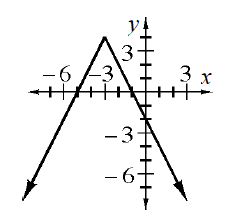### Home > INT3 > Chapter 3 > Lesson 3.1.3 > Problem3-53

3-53.

Use your knowledge of absolute value functions to write the equation of the graph below.What shape is the graph?
Which direction does it open?
Where is the vertex?
How 'steep' is the graph?

$y=-2\left | x+3 \right |+4$